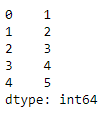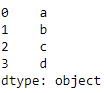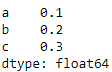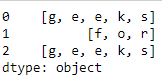Related Articles

# Create and display a one-dimensional array-like object using Pandas in Python

• Last Updated : 18 Aug, 2020

Series() is a function present in the Pandas library that creates a one-dimensional array and can hold any type of objects or data in it. In this article, let us learn the syntax, create and display one-dimensional array-like object containing an array of data using Pandas library.

## pandas.Series()

Syntax : pandas.Series(parameters)
Parameters :

• data : Contains data stored in Series.
• index : Values must be hashable and have the same length as data.
• dtype : Data type for the output Series.
• name : The name to give to the Series.
• copy : Copy input data.

Returns : An object of class Series

Example 1 : Creating Series from a list

 `# import the library``import` `pandas as pd`` ` `# create the one-dimensional array``data ``=` `[``1``, ``2``, ``3``, ``4``, ``5``]`` ` `# create the Series``ex1 ``=` `pd.Series(data)`` ` `# displaying the Series``print``(ex1)`

Output :Example 2 :Creating a Series from a NumPy array.

 `# import the pandas and numpy library``import` `pandas as pd``import` `numpy as np`` ` `# create numpy array``data ``=` `np.array([``'a'``, ``'b'``, ``'c'``, ``'d'``])`` ` `# create one-dimensional data``s ``=` `pd.Series(data)`` ` `# display the Series``print``(s)`

Output :Example 3: Creating a Series from a dictionary.

 `# import the pandas library``import` `pandas as pd`` ` `# create dictionary``dict` `=` `{``'a'` `: ``0.1``, ``'b'` `: ``0.2``, ``'c'` `: ``0.3``}`` ` `# create one-dimensional data``s ``=` `pd.Series(``dict``)`` ` `# display the Series``print``(s)`

Output :Example 4 :Creating a Series from list of lists.

 `# importing the module``import` `pandas as pd`` ` `# creating the data``data ``=` `[[``'g'``, ``'e'``, ``'e'``, ``'k'``, ``'s'``],``        ``[``'f'``, ``'o'``, ``'r'``],``        ``[``'g'``, ``'e'``, ``'e'``, ``'k'``, ``'s'``]]`` ` `# creating a Pandas series of lists``s ``=` `pd.Series(data)`` ` `# displaying the Series``print``(s)`

Output :Attention geek! Strengthen your foundations with the Python Programming Foundation Course and learn the basics.

To begin with, your interview preparations Enhance your Data Structures concepts with the Python DS Course. And to begin with your Machine Learning Journey, join the Machine Learning – Basic Level Course

My Personal Notes arrow_drop_up